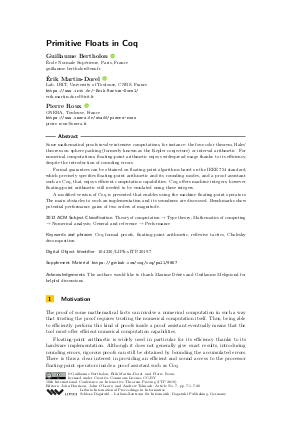Document# Primitive Floats in Coq

### Authors Guillaume Bertholon, Érik Martin-Dorel, Pierre Roux## File

LIPIcs.ITP.2019.7.pdf
• Filesize: 0.6 MB
• 20 pages

## Acknowledgements

The authors would like to thank Maxime Dénès and Guillaume Melquiond for helpful discussions.

## Cite As

Guillaume Bertholon, Érik Martin-Dorel, and Pierre Roux. Primitive Floats in Coq. In 10th International Conference on Interactive Theorem Proving (ITP 2019). Leibniz International Proceedings in Informatics (LIPIcs), Volume 141, pp. 7:1-7:20, Schloss Dagstuhl - Leibniz-Zentrum für Informatik (2019)
https://doi.org/10.4230/LIPIcs.ITP.2019.7

## Abstract

Some mathematical proofs involve intensive computations, for instance: the four-color theorem, Hales' theorem on sphere packing (formerly known as the Kepler conjecture) or interval arithmetic. For numerical computations, floating-point arithmetic enjoys widespread usage thanks to its efficiency, despite the introduction of rounding errors. Formal guarantees can be obtained on floating-point algorithms based on the IEEE 754 standard, which precisely specifies floating-point arithmetic and its rounding modes, and a proof assistant such as Coq, that enjoys efficient computation capabilities. Coq offers machine integers, however floating-point arithmetic still needed to be emulated using these integers. A modified version of Coq is presented that enables using the machine floating-point operators. The main obstacles to such an implementation and its soundness are discussed. Benchmarks show potential performance gains of two orders of magnitude.

## Subject Classification

##### ACM Subject Classification
• Theory of computation → Type theory
• Mathematics of computing → Numerical analysis
• General and reference → Performance
##### Keywords
• Coq formal proofs
• floating-point arithmetic
• reflexive tactics
• Cholesky decomposition

## Metrics

• Access Statistics
• Total Accesses (updated on a weekly basis)
0